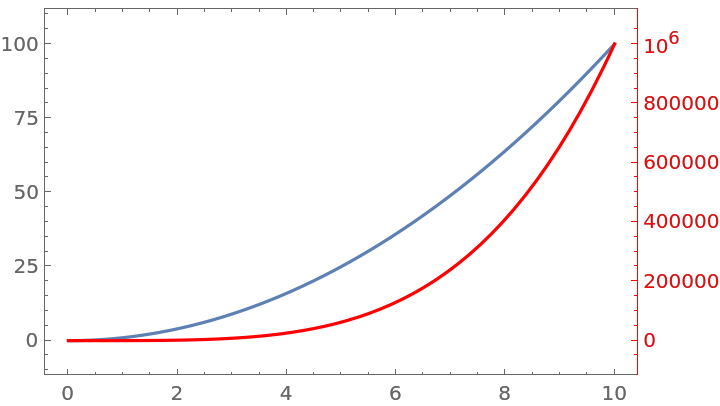#Function Repository Resource:

# CombinePlots

Combine plots, enabling the creation of plots with two sets of axes and merging of prologs and epilogs

Contributed by: Lukas Lang
 ResourceFunction["CombinePlots"][g1,g2,…] works like Show, but can reorder labels/callouts and reposition frame axes.

## Details and Options

ResourceFunction["CombinePlots"] combines graphics expressions while trying to move labels and callouts to the front.
ResourceFunction["CombinePlots"] by default merges the primitives inside the Prolog and Epilog options.
ResourceFunction["CombinePlots"] can reposition frame axes, allowing the creation of plots with two x/y axes.
ResourceFunction["CombinePlots"] accepts the following options:
 "CombineProlog" whether to merge prologs "CombineEpilog" whether to merge epilogs "AnnotationPattern" ()[___] the pattern used to match annotations "AxesSides" on which side to put the frame axes
ResourceFunction["CombinePlots"] effectively removes all expressions matching the pattern specified by "AnnotationPattern" and inserts them on top of the remaining primitives.
The default setting of "AnnotationPattern", ()[___] matches the primitives generated from Label and Callout directives.
The option "AxesSides" accepts the following settings:
 do not move frame axes "TwoY" move the vertical frame axis of the second plot to the right "TwoX" move the horizontal frame axis of the second plot to the top "TwoXY" move the bottom/left frame axes of the second plot to the top/right spec use explicit settings for different plots
"AxesSides" supports the same sequence specification as documented for Spacings.
For each plot, the setting can be one of the following:
 put horizontal frame axis to the bottom put horizontal frame axis to the top put vertical frame axis to the left put vertical frame axis to the right { side x , side y } position both horizontal and vertical frame axes [ side x , side y ] same as {sidex,sidey}
The option "AxesSides" settings "TwoY"/"TwoX"/"TwoXY" are effectively equivalent to settings of the form {2spec}.
The sides for the frame axes of plots can also be specified by wrapping plots with Axes[plot,sideSpec], similar to how Item works for Grid.
The arguments of ResourceFunction["CombinePlots"] can be (nested) lists of plots, where each level can be wrapped in Axes[plots,sideSpec]. Deeper specifications override outer ones as necessary.
The setting of "AxesSides" applies to each argument of ResourceFunction["CombinePlots"] as one. Use Axes[] type specifications for more granular control.
ResourceFunction["CombinePlots"] will use the frame axis of the first appropriate plot for each side. The values of the FrameLabel, FrameTicks, FrameStyle and FrameTicksStyle options are used to create the frame axis in the resulting plot.
ResourceFunction["CombinePlots"] effectively uses GeometricTransformation to rescale plot contents shown on secondary axes.
ResourceFunction["CombinePlots"] sets CoordinatesToolOptions to enable extraction of coordinates from any of the axes. The format is {{sidex,sidey}{x,y},}, with one entry for each unique horizontal/vertical axis combination.
ResourceFunction["CombinePlots"] will always return a (possibly Legended) Graphics expression.
If there are no labels/callouts and no prolog/epilog to combine, ResourceFunction["CombinePlots"][args] is equivalent to Show[args].

## Examples

### Basic Examples (3)

Combine two plots containing annotations:

 In:=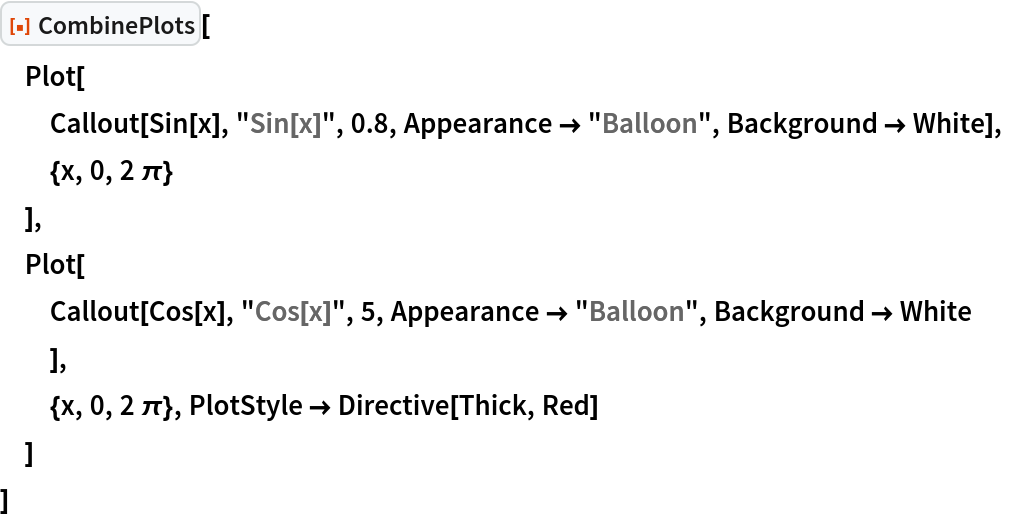Out=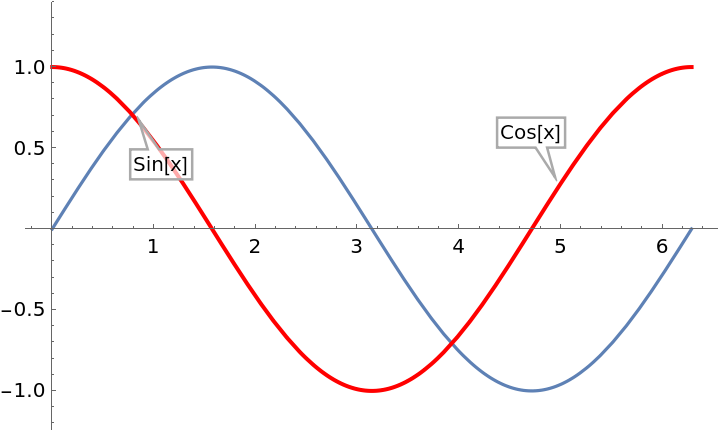If Show is used instead, the annotations of the first plot are covered by the contents of the second one:

 In:=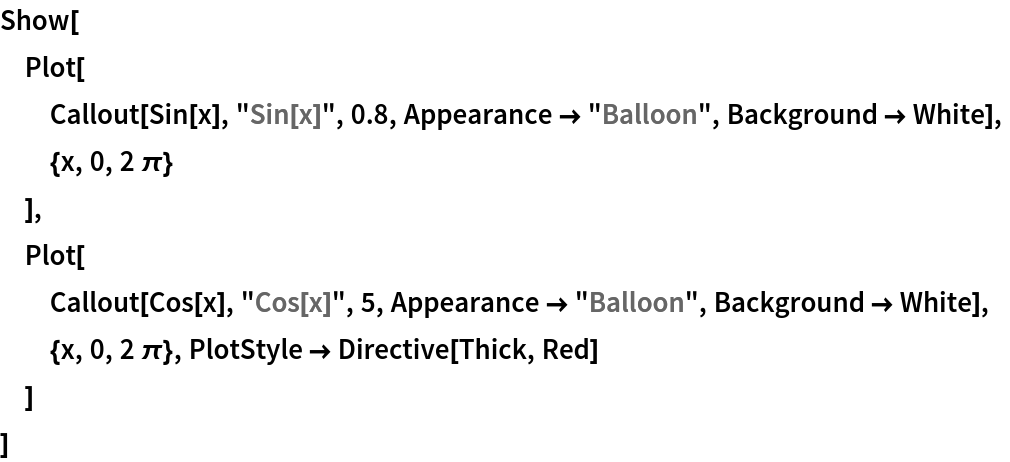Out=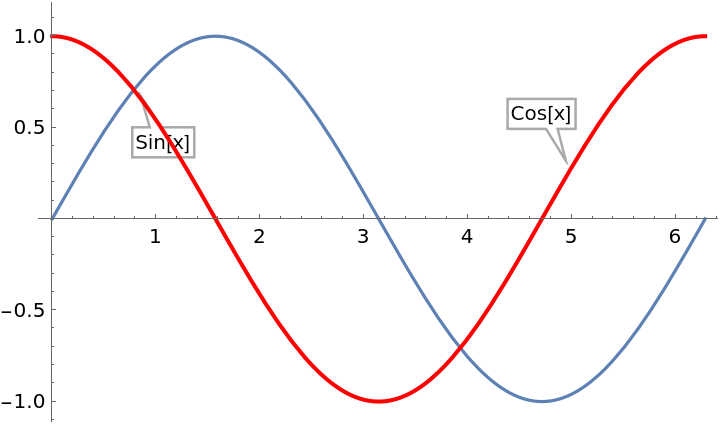Use CombinePlots to combine Prolog and Epilog contents:

 In:=Out=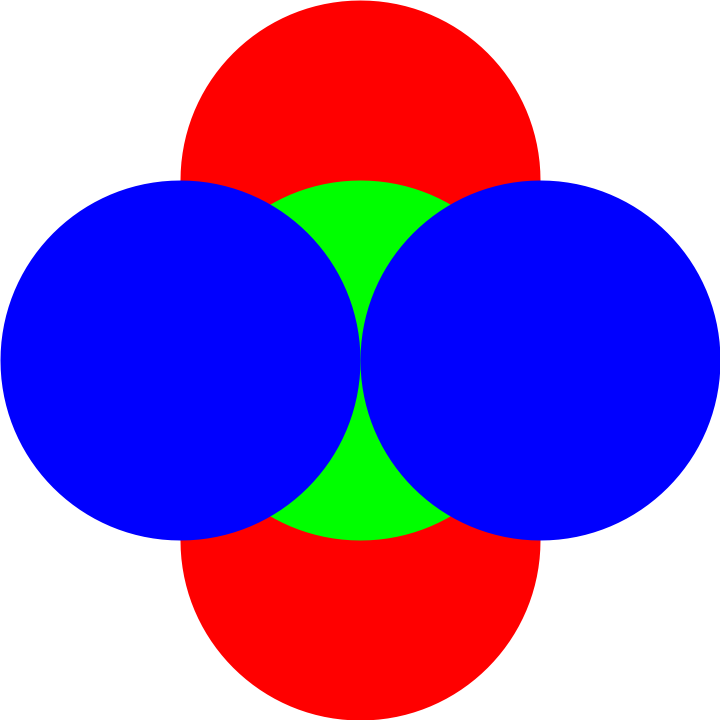Show only keeps the Prolog/Epilog of the first Graphics:

 In:=Out=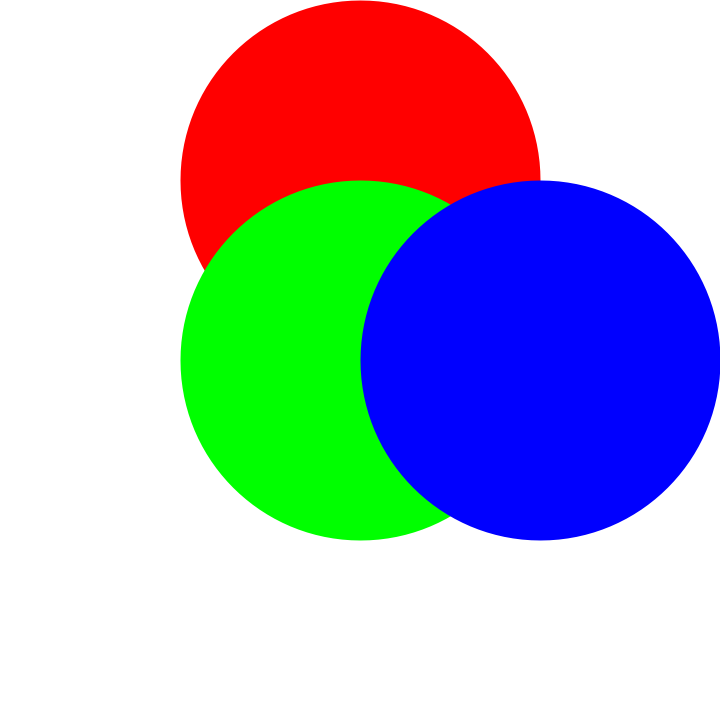Use CombinePlots to create a plot with two vertical axes:

 In:=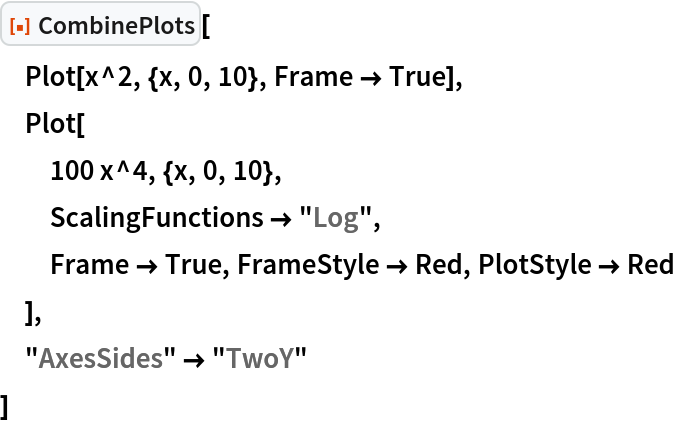Out=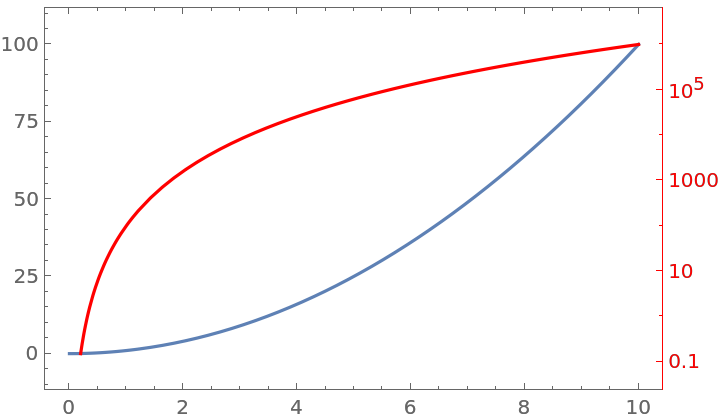### Scope (1)

Merge arbitrary plots into plots with two y axes:

 In:=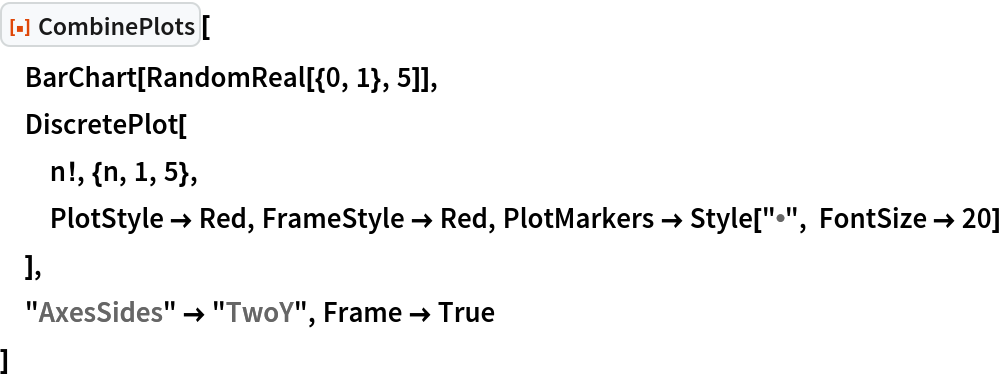Out=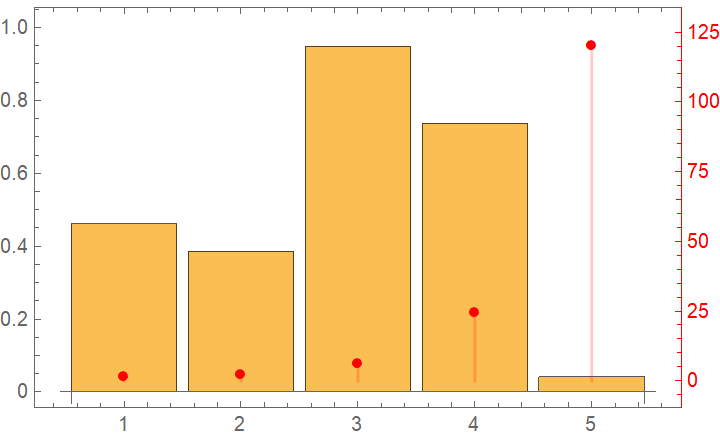### Options (16)

#### CombineProlog (2)

With the default setting "CombineProlog"True, the graphics primitives of each Prolog are combined:

 In:=Out=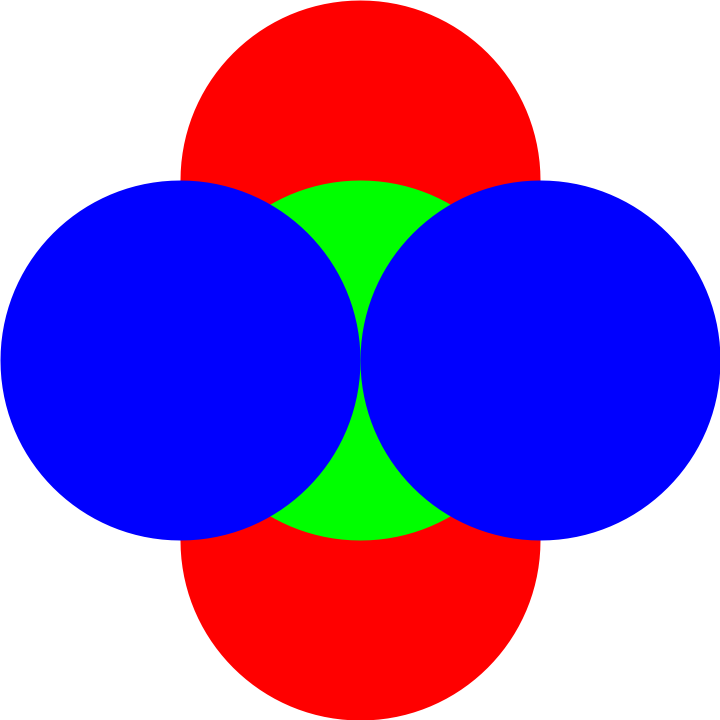Only take the Prolog of the first Graphics, mimicking the behavior of Show:

 In:=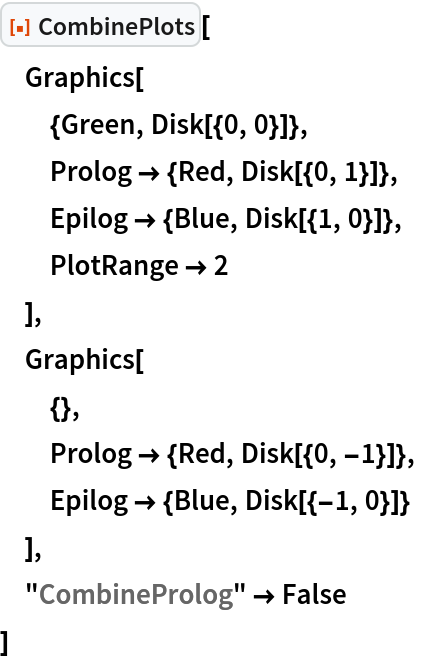Out=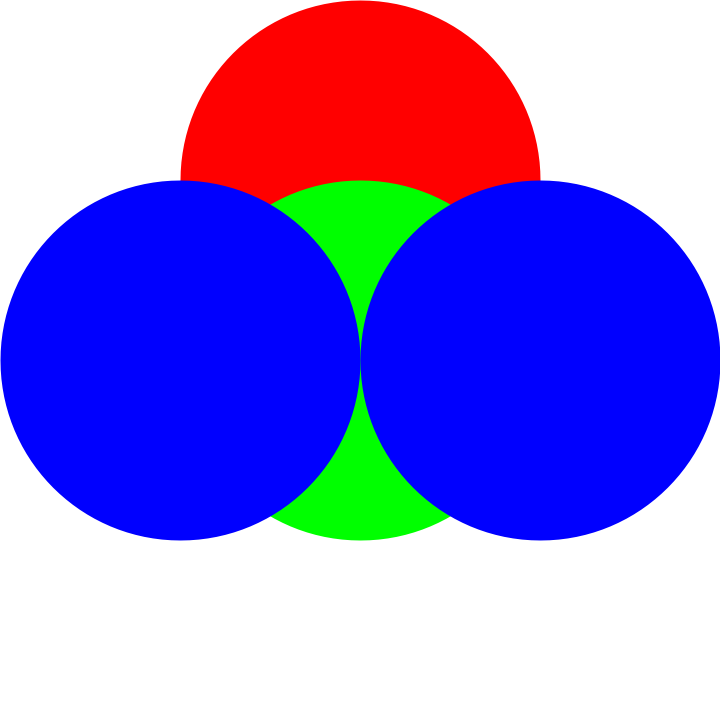#### CombineEpilog (2)

With the default setting "CombineEpilog"True, the graphics primitives of each Epilog are combined:

 In:=Out=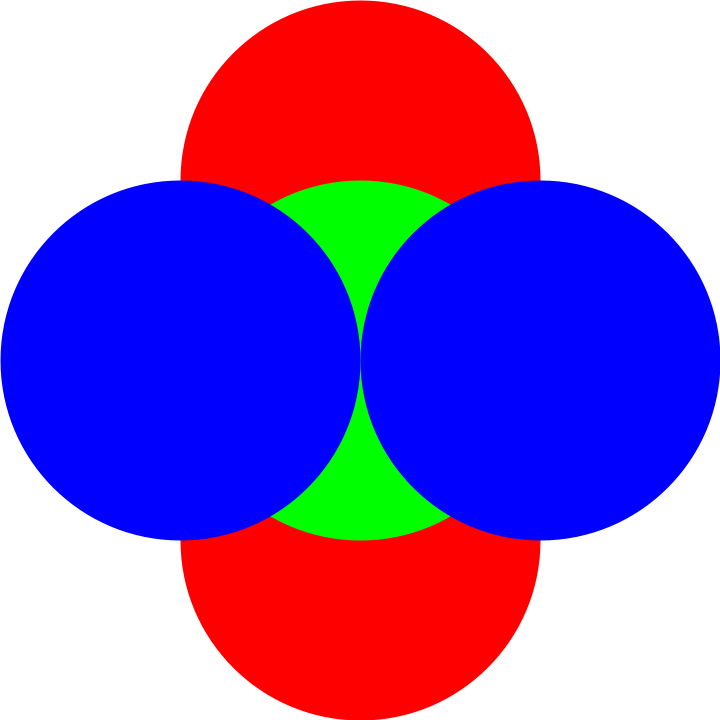Only take the Epilog of the first Graphics, mimicking the behavior of Show:

 In:=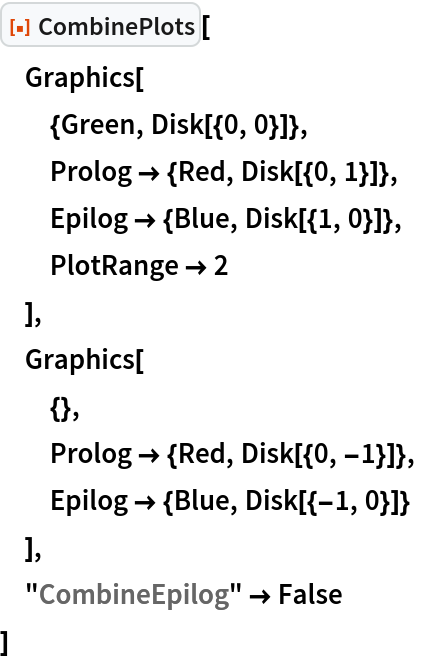Out=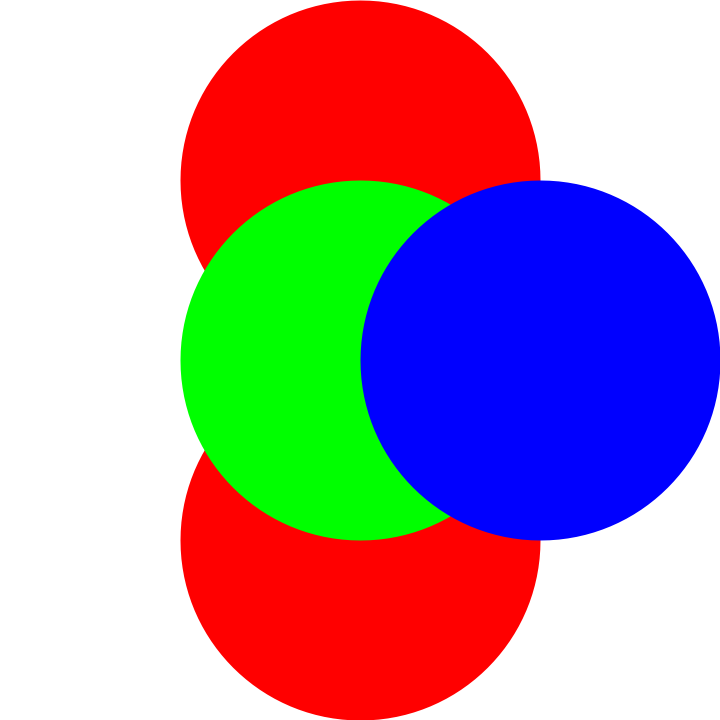#### AnnotationPattern (3)

The default setting of "AnnotationPattern" matches primitives generated for Callout and Label wrappers:

 In:=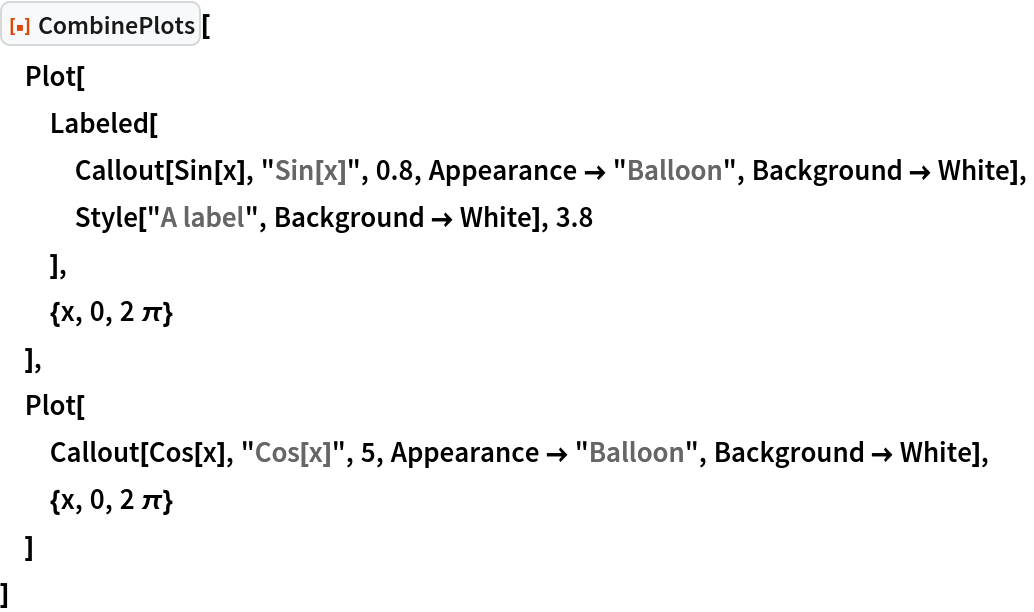Out=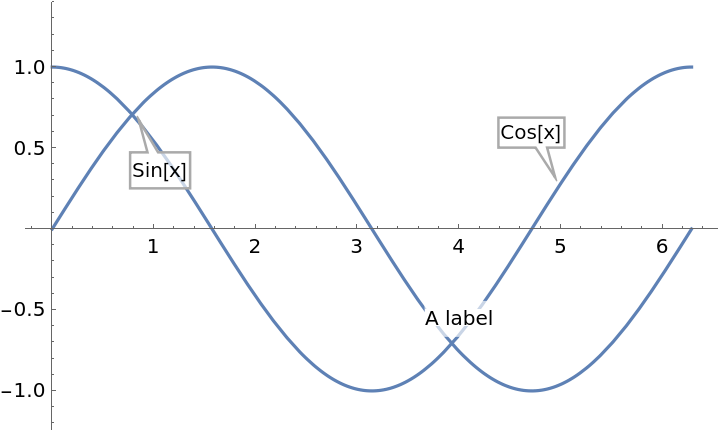Bring only Text primitives to the front:

 In:=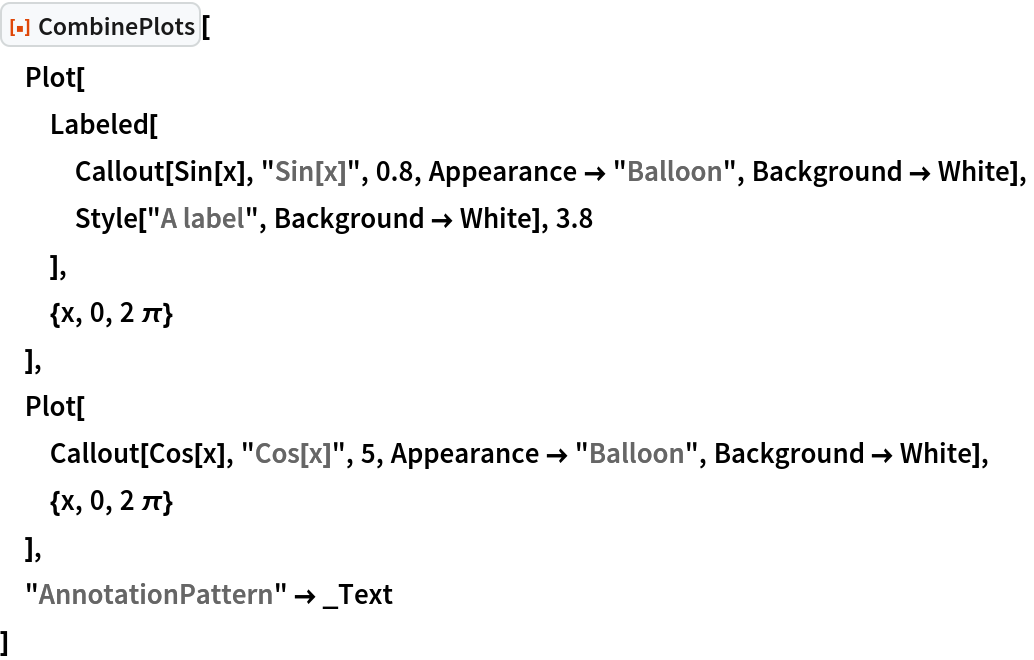Out=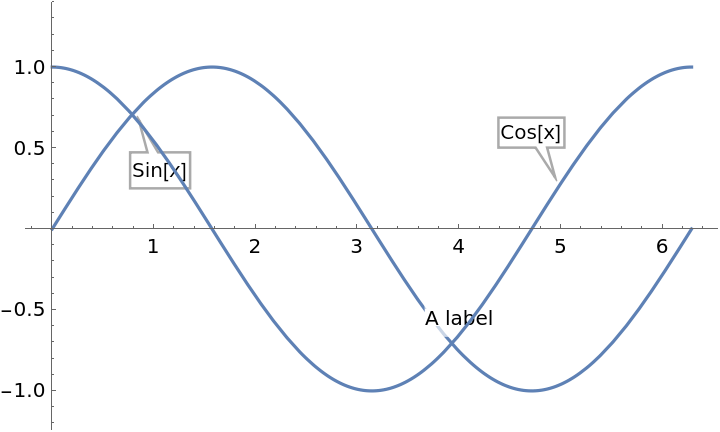Bring only GraphicsGroup primitives to the front:

 In:=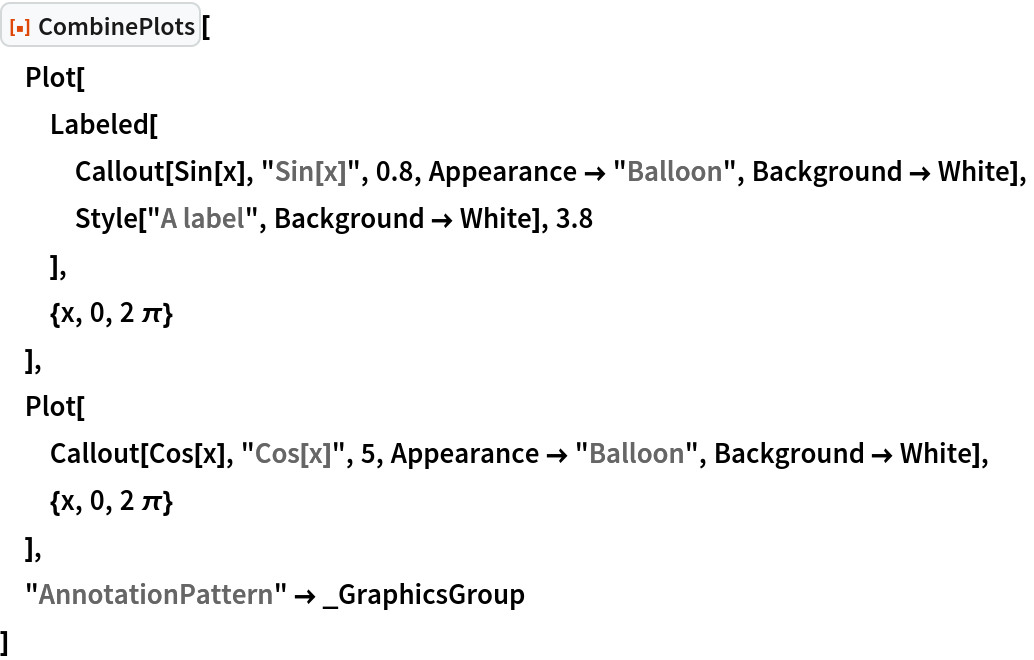Out=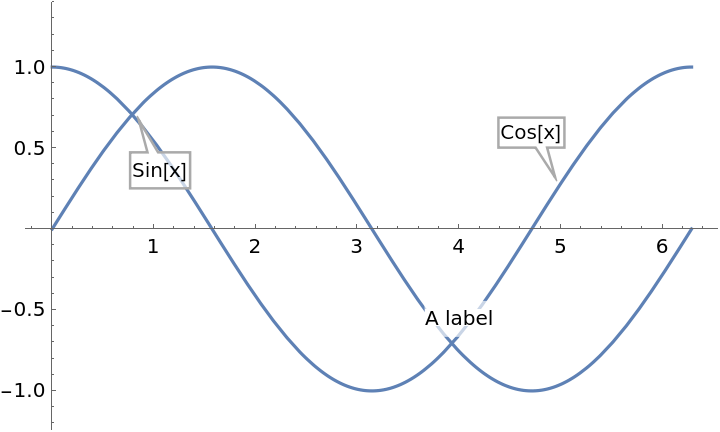#### AxesSides (9)

With the default setting "AxesSides"Automatic, all plots share the same axes:

 In:=Out=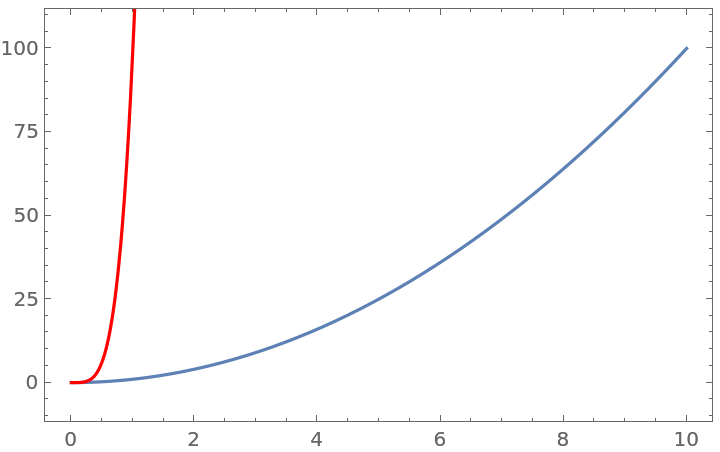Use a secondary vertical axis for the second plot:

 In:=Out=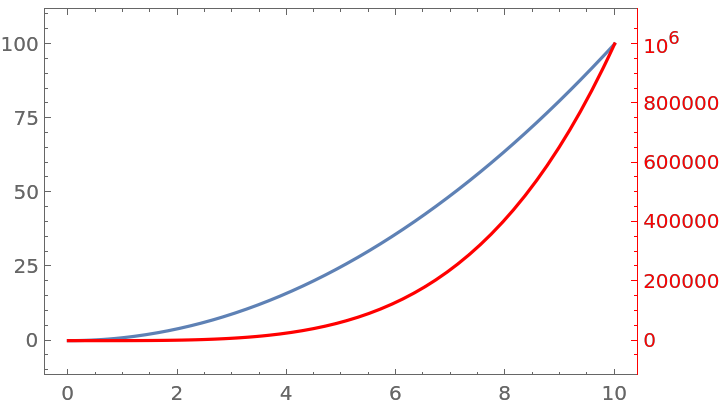The different axes can have different ScalingFunctions:

 In:=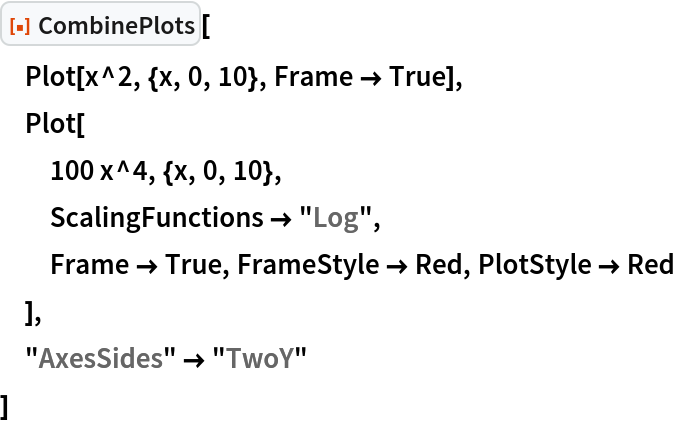Out=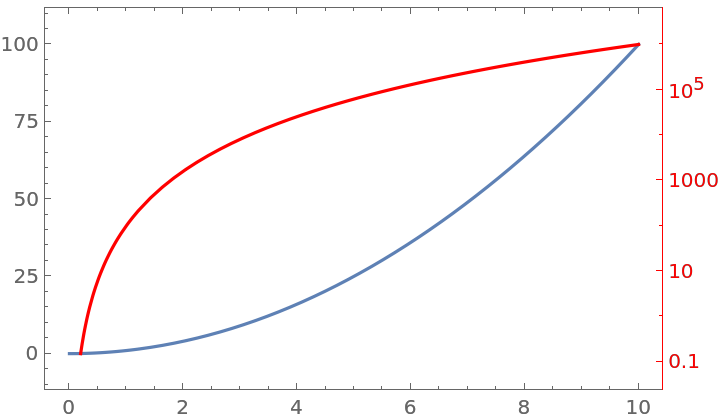Create a plot with a secondary horizontal axis:

 In:=Out=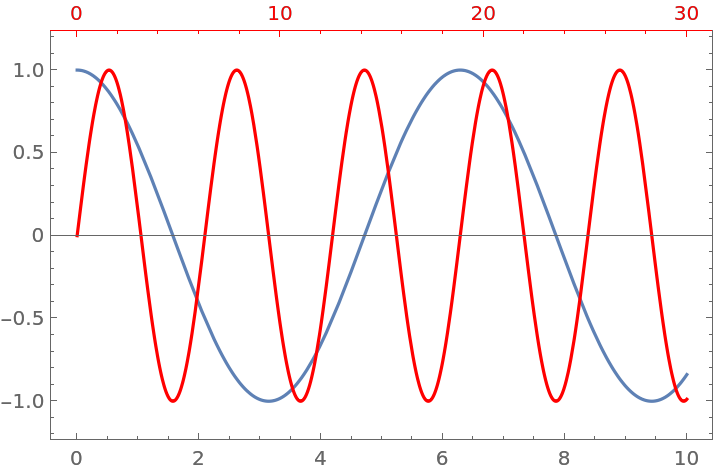Use both secondary x and y axes:

 In:=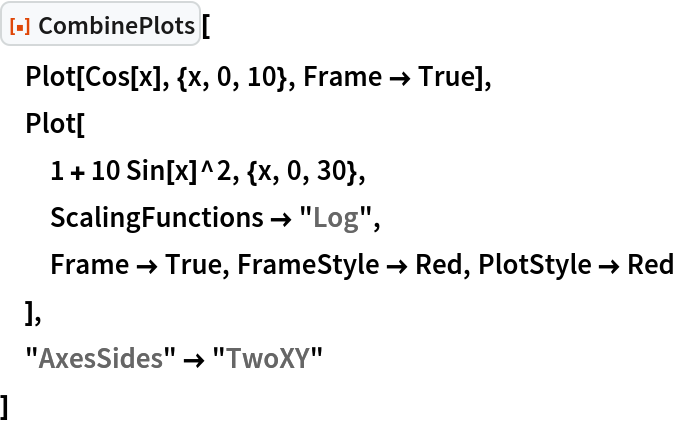Out=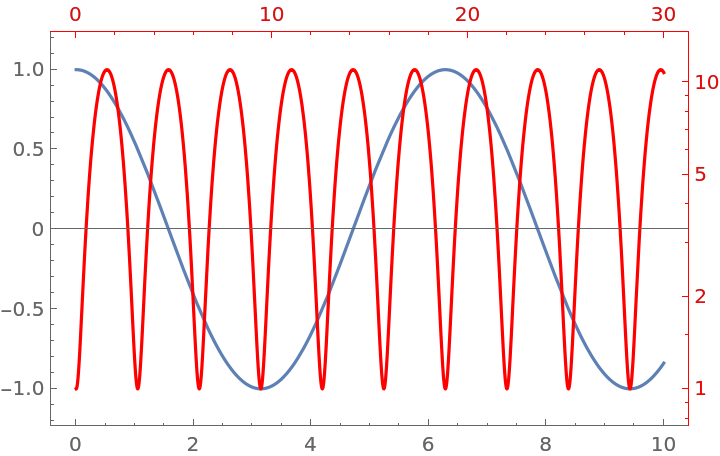Put the contents of multiple plots on the secondary axis:

 In:=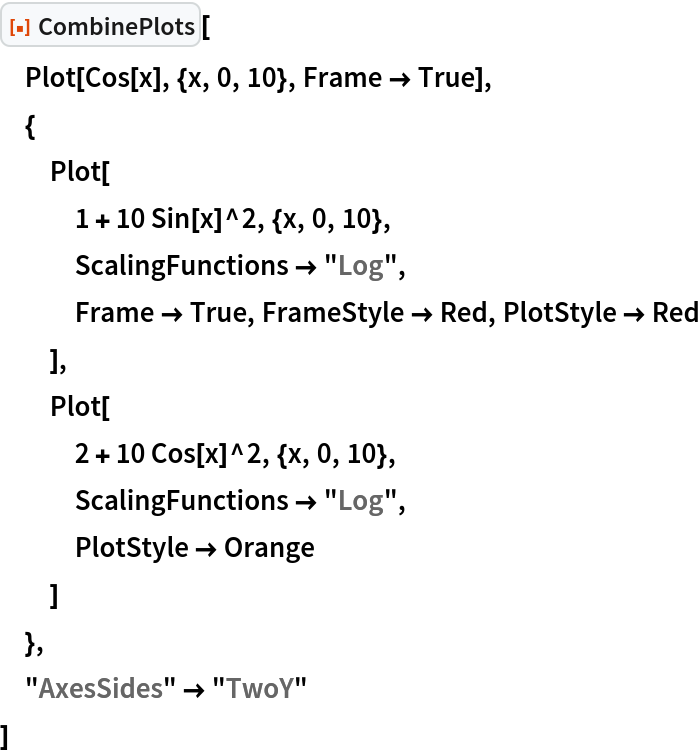Out=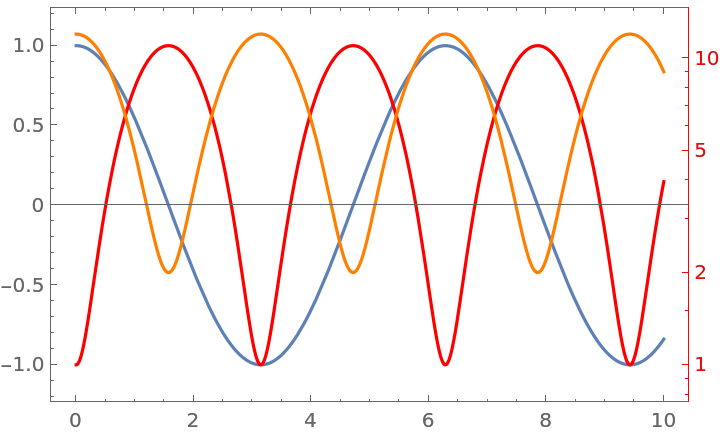Move the vertical axis of all plots to the right:

 In:=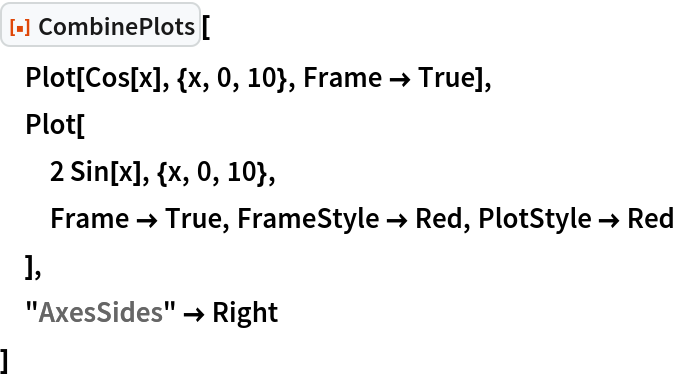Out=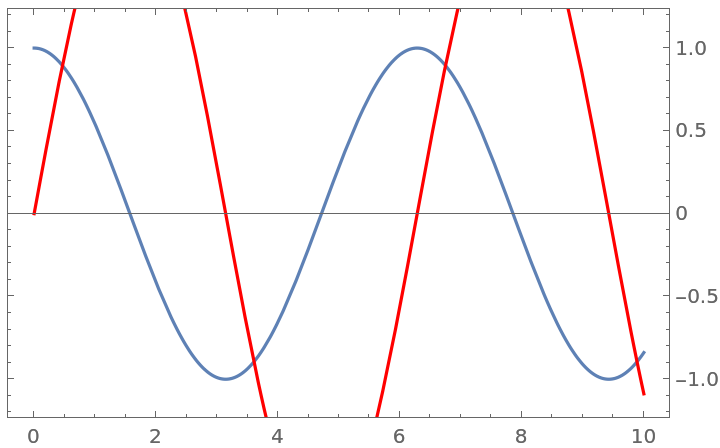Use Axes[plot,sideSpec] to specify the sides on which to put axes:

 In:=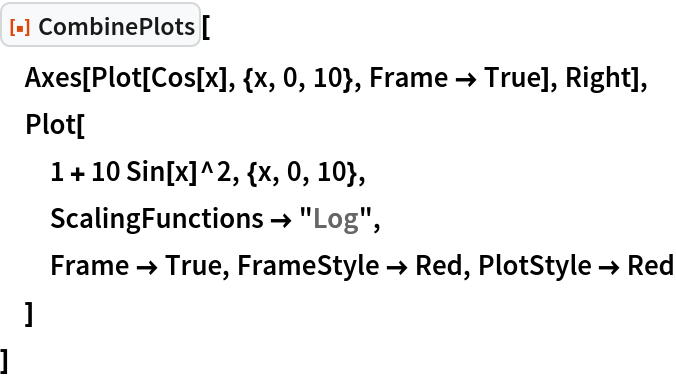Out=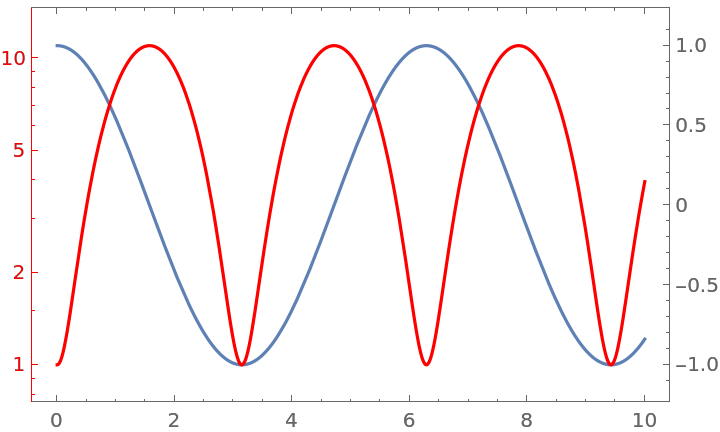Put one plot on the secondary vertical axis, and another one on both the secondary horizontal and vertical axes:

 In:=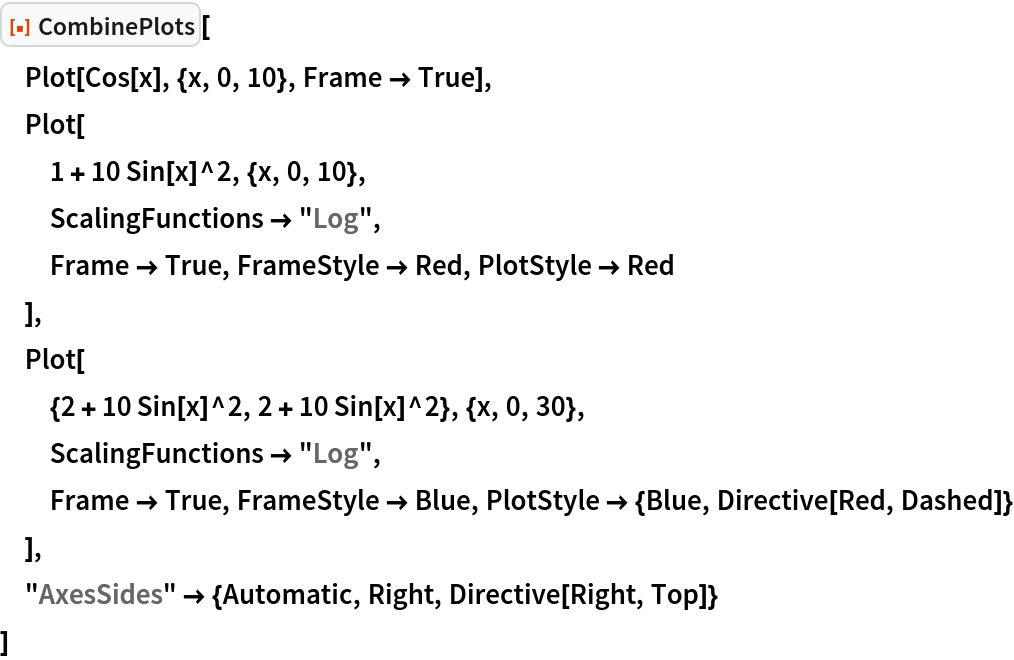Out=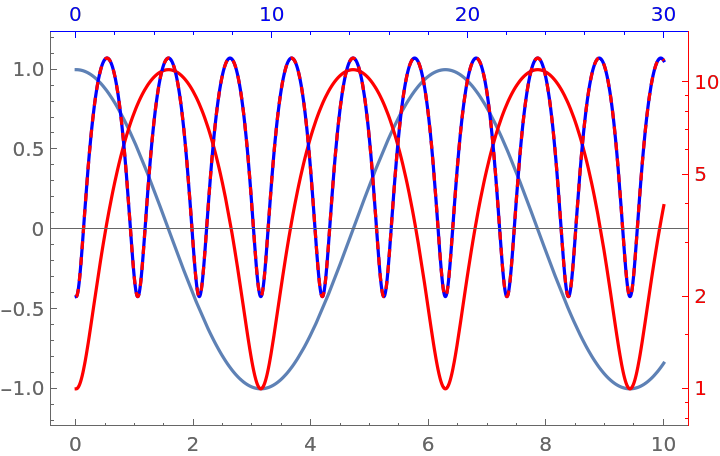### Possible Issues (2)

CombinePlots only moves the Frame type axis. Normal Axes are not supported:

 In:=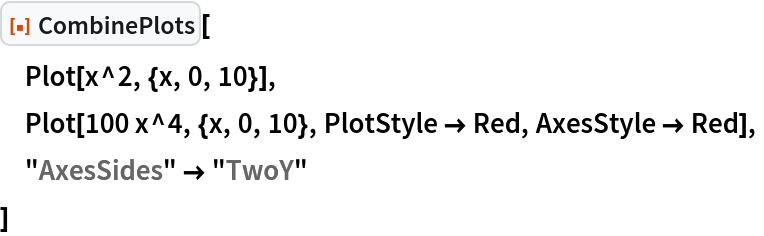Out=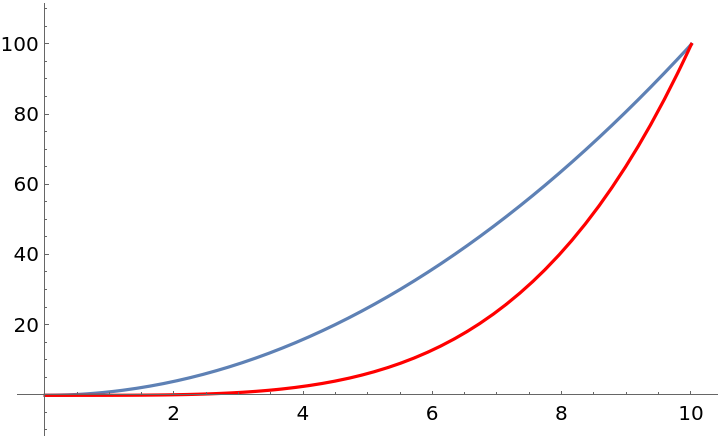Specify to fix the issue:

 In:=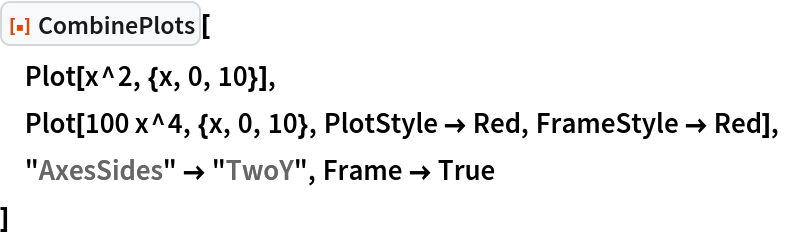Out=